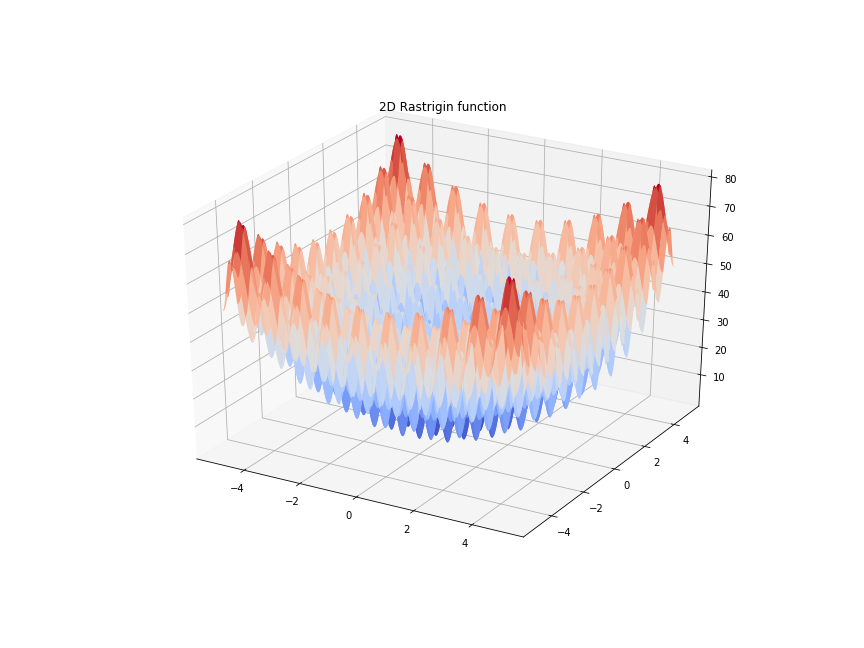# DifferentialEvolutionDriver#

Note

DifferentialEvolutionDriver is based on SimpleGADriver and supports most of the same options and capabilities.

This differential evolution variant of a genetic algorithm optimizer supports only continuous variables. The DifferentialEvolutionDriver supports both constrained and unconstrained optimization.

• Pros

• DifferentialEvolutionDriver is usually more accurate than SimpleGADriver because it does not limit the number of bits available to represent inputs

• DifferentialEvolutionDriver does not require the user to manually specify a number of representation bits

• Cons

• DifferentialEvolutionDriver only supports continuous input variables; SimpleGADriver also supports discrete

• DifferentialEvolutionDriver does not support SimpleGADriver’s “compute_pareto” option for multi-objective optimization

Genetic algorithms do not use gradient information to find optimal solutions. This makes them ideal for problems that do not have gradients or problems with many local minima where gradient information is not helpful in finding the global minimum. A well known example of this is finding the global minimum of of the Rastrigin function:The example below shows an OpenMDAO solution of a higher order Rastrigin function.

import openmdao.api as om
import numpy as np

ORDER = 6  # dimension of problem
span = 5   # upper and lower limits

class RastriginComp(om.ExplicitComponent):
def setup(self):

def compute(self, inputs, outputs):
x = inputs['x']

# nth dimensional Rastrigin function, array input and scalar output
# global minimum at f(0,0,0...) = 0
n = len(x)
s = 10 * n
for i in range(n):
if np.abs(x[i]) < 1e-200:  # avoid underflow runtime warnings from squaring tiny numbers
x[i] = 0.0
s += x[i] * x[i] - 10 * np.cos(2 * np.pi * x[i])

outputs['y'] = s

prob = om.Problem()

lower=-span * np.ones(ORDER),
upper=span * np.ones(ORDER))

prob.driver = om.DifferentialEvolutionDriver()
prob.driver.options['max_gen'] = 400
prob.driver.options['Pc'] = 0.5
prob.driver.options['F'] = 0.5

prob.setup()
prob.run_driver()

print(prob['rastrigin.y'])
print(prob['x'])

[fv-az520-749:45113] mca_base_component_repository_open: unable to open mca_btl_openib: librdmacm.so.1: cannot open shared object file: No such file or directory (ignored)

/usr/share/miniconda/envs/test/lib/python3.11/site-packages/openmdao/core/group.py:864: DerivativesWarning:Constraints or objectives [rastrigin.y] cannot be impacted by the design variables of the problem because no partials were defined for them in their parent component(s).

[0.]
[0. 0. 0. 0. 0. 0.]


## DifferentialEvolutionDriver Options#

OptionDefaultAcceptable ValuesAcceptable TypesDescription
Pc0.9N/AN/ACrossover probability.
debug_print[]['desvars', 'nl_cons', 'ln_cons', 'objs', 'totals']['list']List of what type of Driver variables to print at each iteration.
invalid_desvar_behaviorwarn['warn', 'raise', 'ignore']N/ABehavior of driver if the initial value of a design variable exceeds its bounds. The default value may beset using the OPENMDAO_INVALID_DESVAR_BEHAVIOR environment variable to one of the valid options.
max_gen100N/AN/ANumber of generations before termination.
multi_obj_exponent1.0N/AN/AMulti-objective weighting exponent.
multi_obj_weights{}N/A['dict']Weights of objectives for multi-objective optimization.Weights are specified as a dictionary with the absolute namesof the objectives. The same weights for all objectives are assumed, if not given.
penalty_exponent1.0N/AN/APenalty function exponent.
penalty_parameter10.0N/AN/APenalty function parameter.
pop_size0N/AN/ANumber of points in the GA. Set to 0 and it will be computed as 20 times the total number of inputs.
procs_per_model1N/AN/ANumber of processors to give each model under MPI.
run_parallelFalse[True, False]['bool']Set to True to execute the points in a generation in parallel.

## DifferentialEvolutionDriver Constructor#

The call signature for the DifferentialEvolutionDriver constructor is:

DifferentialEvolutionDriver.__init__(**kwargs)[source]

Initialize the DifferentialEvolutionDriver driver.

## Using DifferentialEvolutionDriver#

You can change the number of generations to run the genetic algorithm by setting the “max_gen” option.

import openmdao.api as om
from openmdao.test_suite.components.branin import Branin

prob = om.Problem()
model = prob.model

model.add_subsystem('comp', Branin(), promotes_inputs=[('x0', 'xI'), ('x1', 'xC')])

prob.driver = om.DifferentialEvolutionDriver()
prob.driver.options['max_gen'] = 5

prob.setup()
prob.run_driver()


You can change the population size by setting the “pop_size” option. The default value for pop_size is 0, which means that the driver automatically computes a population size that is 20 times the total number of input variables.

import openmdao.api as om
from openmdao.test_suite.components.branin import Branin

prob = om.Problem()
model = prob.model

model.add_subsystem('comp', Branin(), promotes_inputs=[('x0', 'xI'), ('x1', 'xC')])